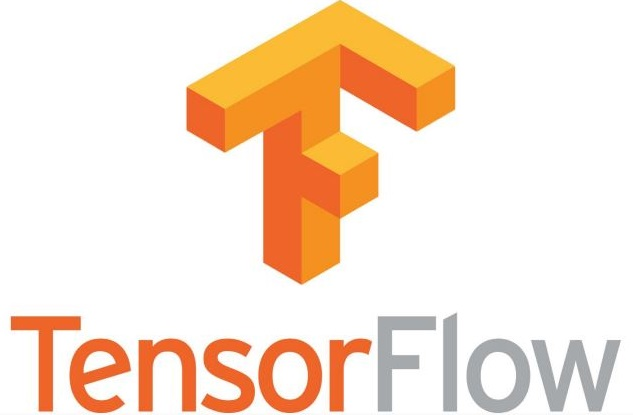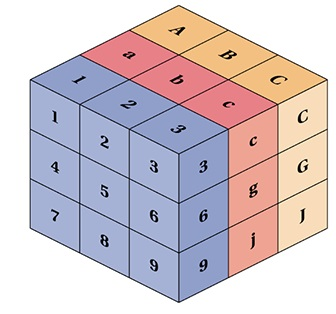# TensorFlow.js Tutorial## What is TensorFlow.js?

Tensorflow is popular JavaScript library for Machine Learning.

Tensorflow lets us train and deploy machine learning in the Browser.

Tensorflow lets us add machine learning functions to any Web Application.

## Using TensorFlow

To use TensorFlow.js, add the following script tag to your HTML file(s):

### Example

<script src="https://cdn.jsdelivr.net/npm/@tensorflow/tfjs@3.6.0/dist/tf.min.js"></script>

If you always want to use the latest version, drop the version number:

### Example 2

<script src="https://cdn.jsdelivr.net/npm/@tensorflow/tfjs"></script>

TensorFlow was developed by the Google Brain Team for internal Google use, but was released as open software in 2015.

In January 2019, Google developers released TensorFlow.js, the JavaScript Implementation of TensorFlow.

Tensorflow.js was designed to provide the same features as the original TensorFlow library written in Python.

## Tensors

TensorFlow.js is a JavaScript library to define and operate on Tensors.

The main data type in TensorFlow.js is the Tensor.

A Tensor is much the same as a multidimensional array.

A Tensor contains values in one or more dimensions:A Tensor has the following main properties:

PropertyDescription
dtypeThe data type
rankThe number of dimensions
shapeThe size of each dimension

Sometimes in machine learning, the term "dimension" is used interchangeably with "rank.

[10, 5] is a 2-dimensional tensor or a 2-rank tensor.

In addition the term "dimensionality" can refer to the size of a one dimension.

Example: In the 2-dimensional tensor [10, 5], the dimensionality of the first dimension is 10.

## Creating a Tensor

The main data type in TensorFlow is the Tensor.

A Tensor is created from any N-dimensional array with the tf.tensor() method:

### Example 1

const myArr = [[1, 2, 3, 4]];
const tensorA = tf.tensor(myArr);

Try it Yourself »

### Example 2

const myArr = [[1, 2], [3, 4]];
const tensorA = tf.tensor(myArr);

Try it Yourself »

### Example 3

const myArr = [[1, 2], [3, 4], [5, 6]];
const tensorA = tf.tensor(myArr);

Try it Yourself »

## Tensor Shape

A Tensor can also be created from an array and a shape parameter:

### Example1

const myArr = [1, 2, 3, 4]:
const shape = [2, 2];
const tensorA = tf.tensor(myArr, shape);

Try it Yourself »

### Example2

const tensorA = tf.tensor([1, 2, 3, 4], [2, 2]);

Try it Yourself »

### Example3

const myArr = [[1, 2], [3, 4]];
const shape = [2, 2];
const tensorA = tf.tensor(myArr, shape);

Try it Yourself »

## Retrieve Tensor Values

You can get the data behind a tensor using tensor.data():

### Example

const myArr = [[1, 2], [3, 4]];
const shape = [2, 2];
const tensorA = tf.tensor(myArr, shape);
tensorA.data().then(data => display(data));

function display(data) {
document.getElementById("demo").innerHTML = data;
}

Try it Yourself »

You can get the array behind a tensor using tensor.array():

### Example

const myArr = [[1, 2], [3, 4]];
const shape = [2, 2];
const tensorA = tf.tensor(myArr, shape);
tensorA.array().then(array => display(array));

function display(data) {
document.getElementById("demo").innerHTML = data;
}

Try it Yourself »

const myArr = [[1, 2], [3, 4]];
const shape = [2, 2];
const tensorA = tf.tensor(myArr, shape);
tensorA.array().then(array => display(array));

function display(data) {
document.getElementById("demo").innerHTML = data;
}

Try it Yourself »

You can get the rank of a tensor using tensor.rank:

### Example

const myArr = [1, 2, 3, 4];
const shape = [2, 2];
const tensorA = tf.tensor(myArr, shape);

document.getElementById("demo").innerHTML = tensorA.rank;

Try it Yourself »

You can get the shape of a tensor using tensor.shape:

### Example

const myArr = [1, 2, 3, 4];
const shape = [2, 2];
const tensorA = tf.tensor(myArr, shape);

document.getElementById("demo").innerHTML = tensorA.shape;

Try it Yourself »

You can get the datatype of a tensor using tensor.dtype:

### Example

const myArr = [1, 2, 3, 4];
const shape = [2, 2];
const tensorA = tf.tensor(myArr, shape);

document.getElementById("demo").innerHTML = tensorA.dtype;

Try it Yourself »

## Tensor Data Types

A Tensor can have the following data types:

• bool
• int32
• float32 (default)
• complex64
• string

When you create a tensor, you can specify the data type as the third parameter:

### Example

const myArr = [1, 2, 3, 4];
const shape = [2, 2];
const tensorA = tf.tensor(myArr, shape, "int32");

Try it Yourself »

W3Schools is optimized for learning and training. Examples might be simplified to improve reading and learning. Tutorials, references, and examples are constantly reviewed to avoid errors, but we cannot warrant full correctness of all content. While using W3Schools, you agree to have read and accepted our terms of use, cookie and privacy policy.